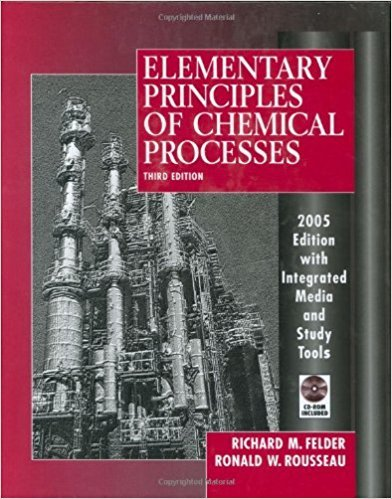×
Get Full Access to Elementary Principles Of Chemical Processes - 3 Edition - Chapter 2 - Problem 2.45
Get Full Access to Elementary Principles Of Chemical Processes - 3 Edition - Chapter 2 - Problem 2.45

×

# The rate at which a substance passes through aISBN: 9780471687573 143

## Solution for problem 2.45 Chapter 2

Elementary Principles of Chemical Processes | 3rd Edition

• Textbook Solutions
• 2901 Step-by-step solutions solved by professors and subject experts
• Get 24/7 help from StudySoup virtual teaching assistantsElementary Principles of Chemical Processes | 3rd Edition

4 5 1 306 Reviews
25
5
Problem 2.45

The rate at which a substance passes through a semipermeable membrane is determined by the diffusivity D(cmZ/s) of the gas. D varies with the membrane temperature T(K) according to the Arrhenius equation: D = Do exp (- E RT) where Do = the preexponential factor E = the activation energy for diffusion R = 1.987 cal/(mol'K) Diffusitivities of SOz in a fluorosilicone rubber tube are measured at several temperatures, with the following results: T(K) 347.0 374.2 396.2 420.7 447.7 471.2 1.34 2.50 4.55 8.52 14.07 19.99 Student Workbook (a) What are the units of Do and E? (b) How should the data be plotted to obtain a straight line on rectangular coordinates? (c) Plot the data in the manner indicated in part (b), and determine Do and E from the resulting line. *(d) Write a computer program or spreadsheet to read in the (T,D) data and to calculate Do and E using the method of least squares (Appendix A.l). Then run the program and print out the results.

Step-by-Step Solution:
Step 1 of 3

Chem 1035 Chapter 12 1 of 30 Chapter 12 Liquids and Solids (Section 12.3 only) Phases of Matter Solids Liquids Gases  Very ordered 3D array  Particles close together,  Container is mostly empty but not ordered space  Doesn’t take shape or fill volume of container  Takes shape, but doesn’t  Gas takes shape and fills  Vibrational motion only fill volume of container volume of container.  Relatively dense  Particles can move past  Particles moving

Step 2 of 3

Step 3 of 3

##### ISBN: 9780471687573

The answer to “The rate at which a substance passes through a semipermeable membrane is determined by the diffusivity D(cmZ/s) of the gas. D varies with the membrane temperature T(K) according to the Arrhenius equation: D = Do exp (- E RT) where Do = the preexponential factor E = the activation energy for diffusion R = 1.987 cal/(mol'K) Diffusitivities of SOz in a fluorosilicone rubber tube are measured at several temperatures, with the following results: T(K) 347.0 374.2 396.2 420.7 447.7 471.2 1.34 2.50 4.55 8.52 14.07 19.99 Student Workbook (a) What are the units of Do and E? (b) How should the data be plotted to obtain a straight line on rectangular coordinates? (c) Plot the data in the manner indicated in part (b), and determine Do and E from the resulting line. *(d) Write a computer program or spreadsheet to read in the (T,D) data and to calculate Do and E using the method of least squares (Appendix A.l). Then run the program and print out the results.” is broken down into a number of easy to follow steps, and 168 words. The full step-by-step solution to problem: 2.45 from chapter: 2 was answered by , our top Chemistry solution expert on 11/15/17, 02:42PM. Since the solution to 2.45 from 2 chapter was answered, more than 302 students have viewed the full step-by-step answer. This full solution covers the following key subjects: data, membrane, program, results, line. This expansive textbook survival guide covers 13 chapters, and 710 solutions. Elementary Principles of Chemical Processes was written by and is associated to the ISBN: 9780471687573. This textbook survival guide was created for the textbook: Elementary Principles of Chemical Processes, edition: 3.

Unlock Textbook Solution